# Chapter 3 Standardizing Data

library(fluoR)
df <- format_data(GCaMP)

## 3.1 Reasons to standardize data

There are a few reasons to standardize your data before exploring your data.

1. Signal differs between subjects

• Regardless of the specific technologies used, there is almost always differences in signal strength for each subject

2. Signal differs between trials

• The strength of recording signal tends to decay over time

3. Utilizing baseline values

• Using transformations such as percent change allows you to center the data at an objective value
• After centering your trial and post-trial data, the data is interpreted as relative to baseline values
• The baseline period is typically assumed to be a “resting” period prior to exposure to the experimental manipulation. This means that using standardization methods (particularly z-scores) also takes baseline deviations into consideration.

## 3.2 Methods of standardization

A little alteration in how we compute z-scores can make a significant difference.

### 3.2.1 z-scores

Standard z-score transformations work the same way with time series data as with any other. The formula:

1. centers every value (x) at the mean of the full time series (mu)
2. divides it by the standard deviation of the full time series (sigma).

$\begin{gather*} z_{i} = \frac{x_{i}-\mu}{\sigma} \end{gather*}$ \begin{align*} \text{where...} \\ \mu &= \text{mean of full trial period,} \\ \sigma &= \text{standard deviation of full trial period} \\ \end{align*}

This results in the same time series in terms of standard deviations from the mean, all in the context of the full time series.

#### 3.2.1.1 R Code

z.scores <- z_score(xvals = df$Trial8, mu = mean(df$Trial8), # manual input of mu/sigma optional;
sigma = sd(dfTrial8)) # used for example purposes #### 3.2.1.2 Visualization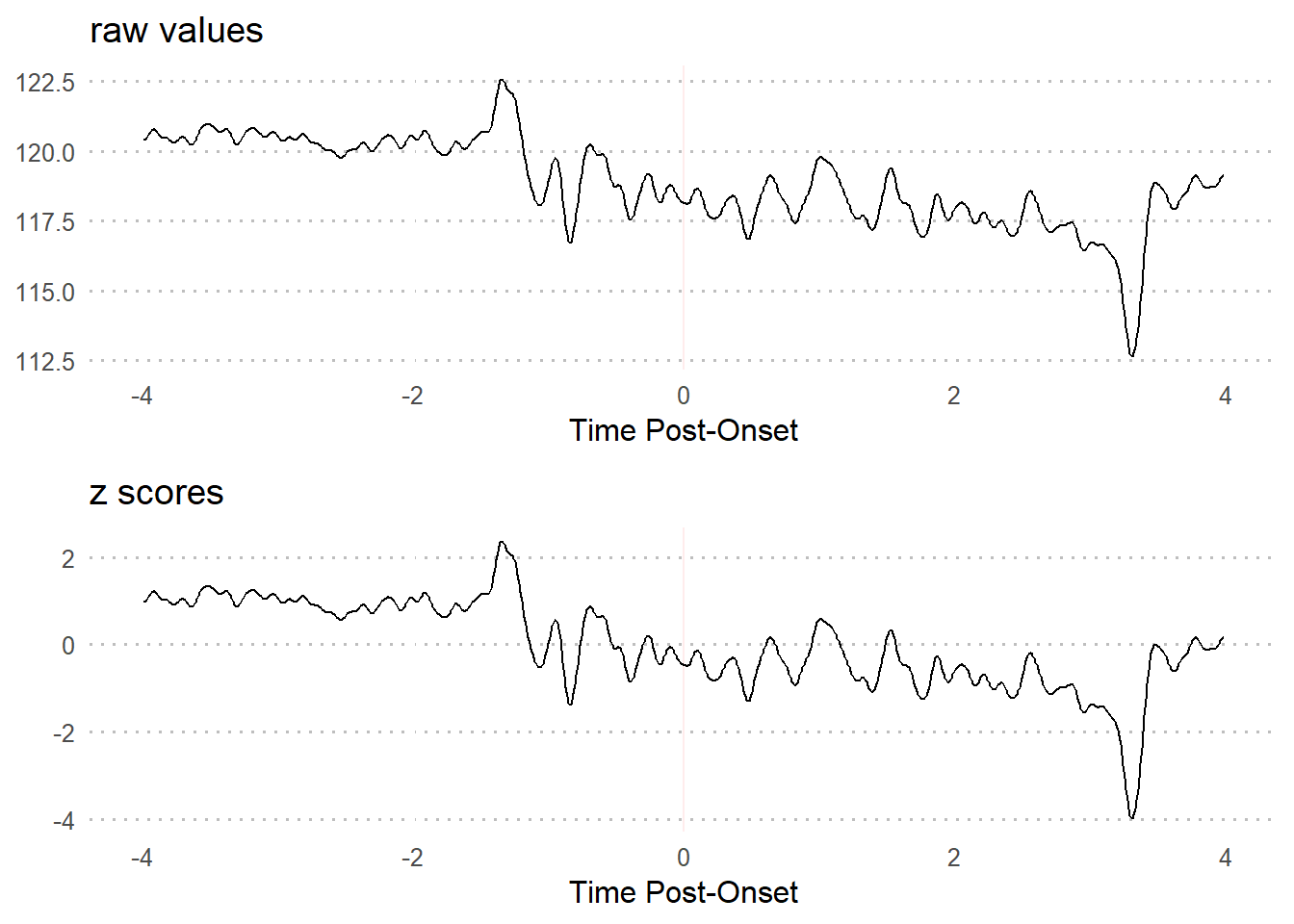### 3.2.2 baseline z-scores Using the pre-event baseline period as the input values for computing z-scores can be useful in revealing changes in neural activity that you may not find by just comparing pre-trial and trial periods. This is in part because baseline periods tend to have relatively low variability. As you can see from the formula, a lower standard deviation will increase positive values and decrease negative values - thus making changes in neural activity more apparent. $\begin{gather*} baseline \ z_{i} = \frac{x_{i}-\bar{x}_{baseline}}{s_{baseline}} \end{gather*}$ \begin{align*} \text{where...} \\ \bar{x}_{baseline} &= \text{mean of values from baseline period,} \\ {s}_{baseline} &= \text{standard deviation of values from baseline period} \\ \end{align*} This results in a time series interpreted in terms of standard deviations and mean during the baseline period. Baseline z-scores are conceptually justifiable because the standard deviation is then the number of deviations from the mean when a subject is at rest. The values outside of the baseline period will be different using this version, but not within the baseline period. #### 3.2.2.1 R Code ### Extract baseline values baseline.vals <- dfTrial8[df$Time >= -4 & df$Time <= 0]

### Compute z-scores
z.scores.baseline <- z_score(xvals = dfTrial8, mu = mean(baseline.vals), sigma = sd(baseline.vals)) #### 3.2.2.2 Visualization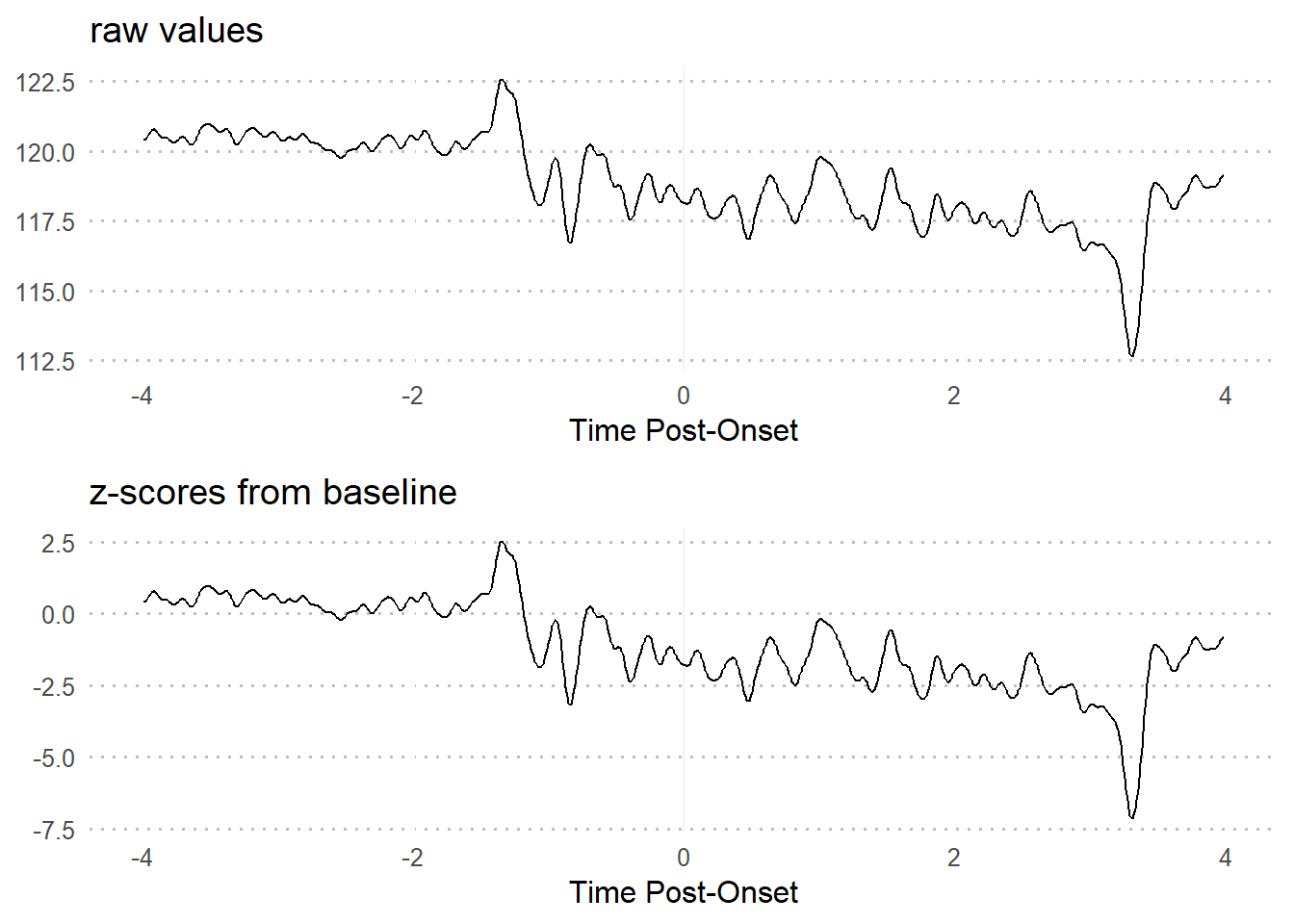### 3.2.3 modified z scores Waveform data fluctuates naturally. But in the event of a change in activity due to external stimuli, signal variation tends to rapidly increase and/or decrease and becomes unruly. $\begin{gather*} modified \ z_{i} = \frac{0.6745(x_{i}-\widetilde{x})}{MAD} \end{gather*}$ \begin{align*} \text{where...} \\ \widetilde{x} &= \text{sample median,} \\ {MAD} &= \text{median absolute deviation} \end{align*} #### 3.2.3.1 R Code z.scores.modified <- z_score(xvals = dfTrial8,
z.type = 'modified')

#### 3.2.3.2 Visualization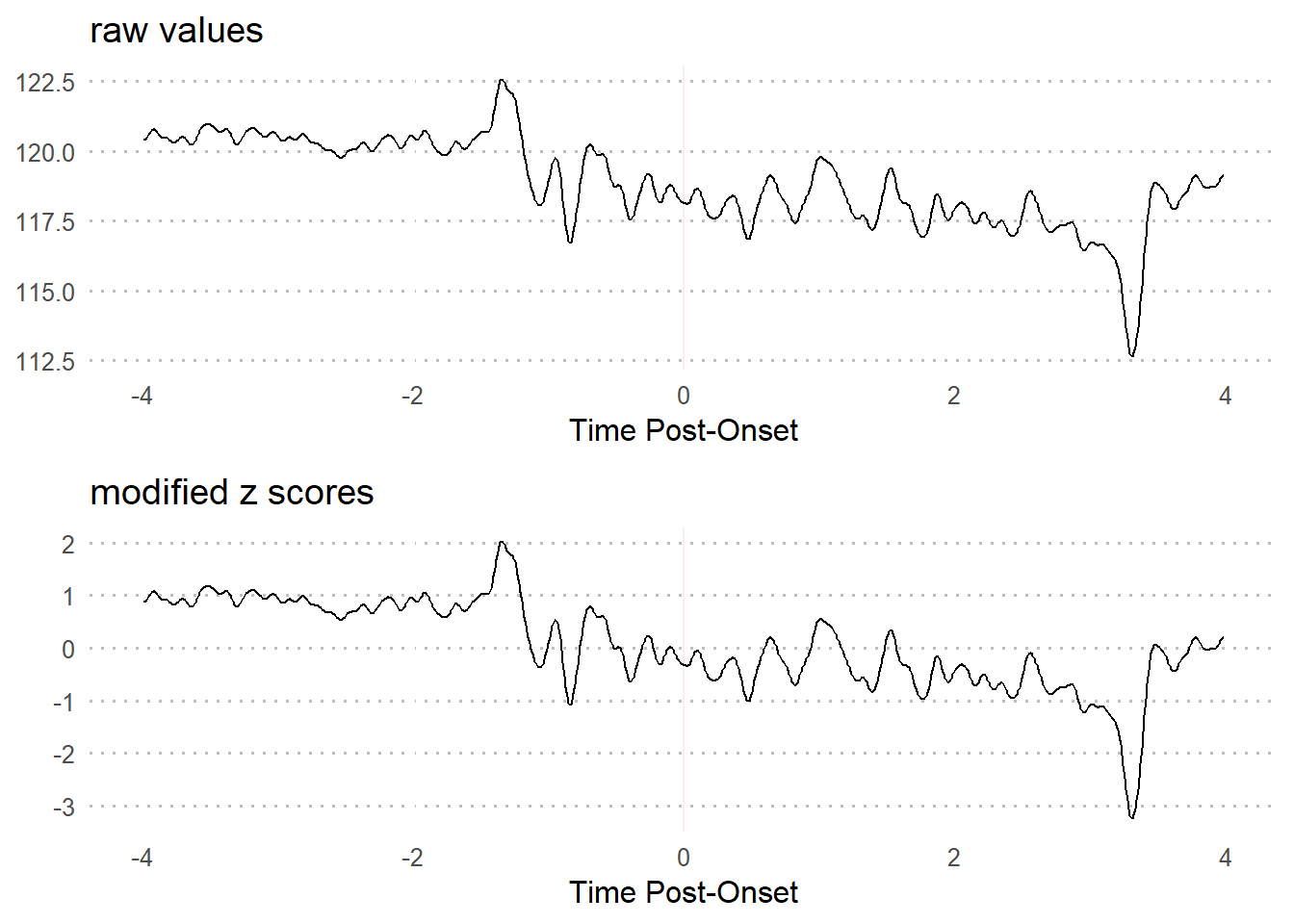## 3.3 z-score comparison

### 3.3.1 Visualization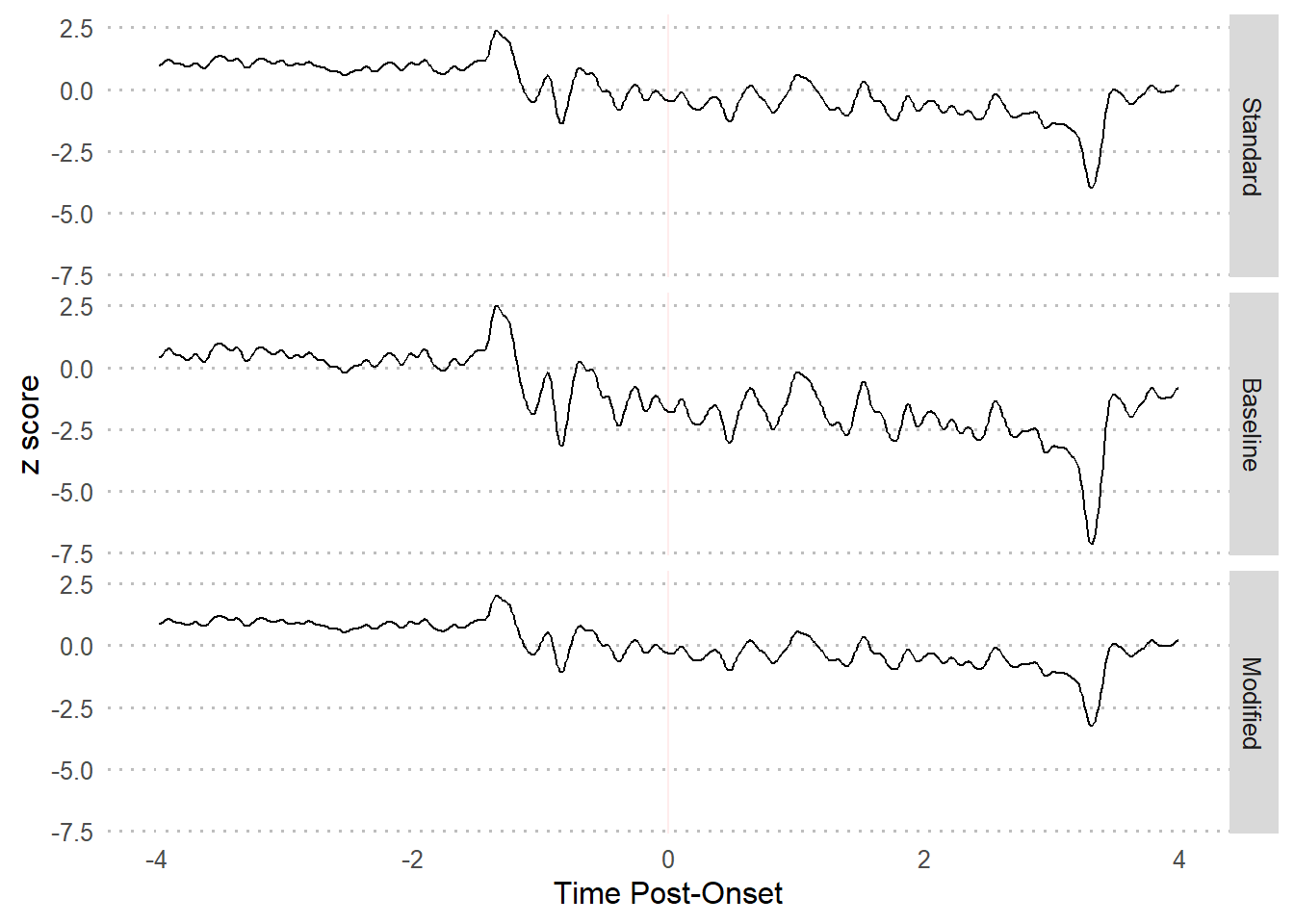### 3.3.2 Summary table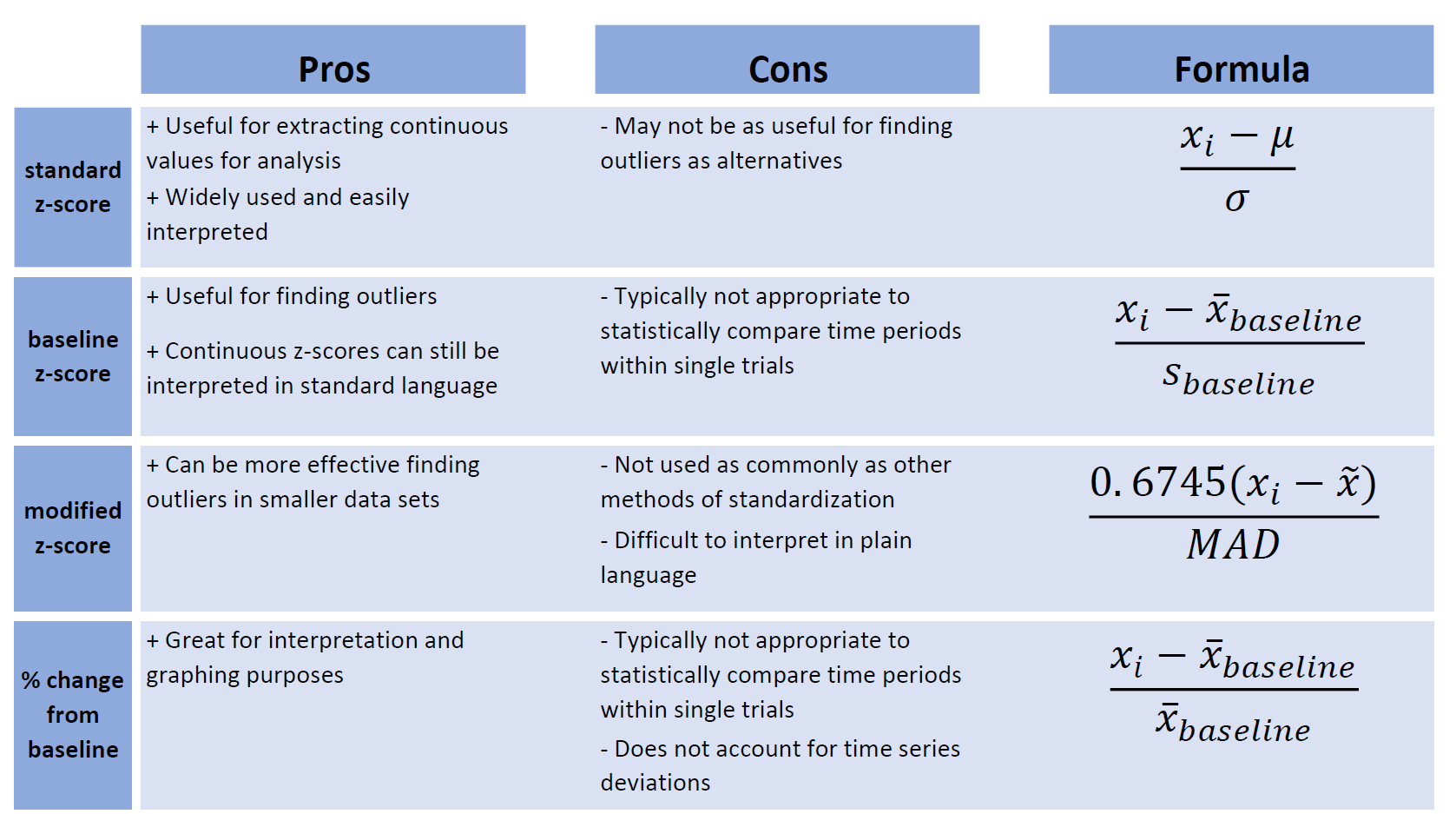## 3.4 Examples

### 3.4.1 Example 1

Standardize trial 8 so that the units are in terms of standard deviations from the mean of the full time series.

z_score(xvals = df$Trial8, z.type = 'standard') ### 3.4.2 Example 2 Standardize trial 8 so that the units are in terms of standard deviations from the mean of the pre-event period. We can do this manually for each trial. ### Manual baseline.vals <- df$Trial8[df$Time >= -4 & df$Time <= 0] # extract baseline values

baseline.z <- z_score(xvals = df$Trial8, mu = mean(baseline.vals), # mean of baseline sigma = sd(baseline.vals), # sd of baseline z.type = 'standard') Or we can use the baseline_transform wrapper. baseline.zdf <- baseline_transform(dataframe = df, trials = 8, baseline.times = c(-4, 0), type = 'z_standard') Both methods will result in the same values. all(baseline.zdf$Trial8 - baseline.z == 0)
##  TRUE

### 3.4.3 Example 3

Standardize trial 8 so that the units are in terms of deviations from the median of the full time series.

z_score(xvals = df$Trial8, z.type = 'modified') ### 3.4.4 Example 4 Convert trial 8 so that the units are in terms of percent change from the mean of the pre-event period. We can do this manually for each trial. ### Manual baseline.vals <- df$Trial8[df$Time >= -4 & df$Time <= 0] # extract baseline values

perc.change <- percent_change(xvals = df$Trial8, base.val = mean(baseline.vals)) Or we can use the baseline_transform wrapper. perc.changedf <- baseline_transform(dataframe = df, trials = 8, baseline.times = c(-4, 0), type = 'percent_change') Both methods will result in the same values. all(perc.changedf$Trial8 - perc.change == 0)
##  TRUE